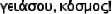#!/usr/local/bin/php

Here are the answers to the JavaScript exercises in the previous sections.

1. Write a JavaScript that outputs the string "Hello, World!" into a web page.
2.  ``````
3. Write a JavaScript that outputs the string "" into a web page (this is the Greek version of #1!).
1. Following the example of the Amherst College Founding, create a web page that calculates how old you are and displays the result.
2.  ```I was born years ago. ``` I was born years ago.
3. Following the example of the College Check, create a web page that uses a form with a text input, providing a number of years in the property `this.value`, and calculate the year when you will be that age. Use the method `window.alert('string')` to display the result.
4.  ```
```

1. For the Spreadsheet Object, modify its special version of toString so that it outputs a form in each cell of the spreadsheet. Then modify the spreadsheet so that an entry in each form changes the corresponding value stored in the spreadsheet. Add a method to check the values in the spreadsheet independently from the form.
2.  ```function c2name(column) {   var name = ''   do {     name = String.fromCharCode(column%26 + 65) + name     column = Math.floor(column/26)   } while (column > 0)   return name } function cr2name() {   var name = c2name(this.column)   name += this.row + 1   return name } function ssToString() {   var ssDisplay = '' +       '
' +         c2name(column) +         '
' + (row + 1) + ''     for (column = 0; column < this.length; column++) {       ssDisplay += '' +             '
'     }   }   ssDisplay += '
'   for (var column = 0; column < this.length; column++) {     ssDisplay += ''   }   for (row = 0; row < this.length; row++) {     ssDisplay += ''   return ssDisplay } function SSCell(column, row, value) {   this.column = column   this.row = row   this.name = cr2name   this.value = value ? value : '' } function SSCol(column, rows) {   var i   var sscol = new Array(rows)   for (i = 0; i < rows; i++) {     sscol[i] = new SSCell(column, i)   }   return sscol } function SSheet(name, columns, rows) {   var i   var ssheet = new Array(columns)   for (i = 0; i < columns; i++) {     ssheet[i] = new SSCol(i, rows)   }   ssheet.name = name   ssheet.toString = ssToString   return ssheet } mySheet = SSheet("mySheet", 2, 2) document.write(mySheet) ``````
`````` ``````

``` ```   This is initially just a paragraph place-holder. ```

A more complete answer would track multiple displays of the spreadsheet, and when one is changed update the forms in the others.

1. Write a JavaScript function that takes one parameter, returns it unchanged if it can't be converted to a number, and otherwise writes it out as a string in "abbreviated" form, using the standard numerical abbreviations:

http://www.bipm.fr/enus/3_SI/si-prefixes.html

Hint: use the base 10 logarithm to calculate the power-of-ten exponent.
2.  Abbreviation:

The power-of-ten exponent of the number can be used as an index into a string to find the appropriate abbreviation:

abbrevLetter = "y..z..a..f..p..n..µ..mcd.DHK..M..G..T..P..E..Z..Y"

 ```

Abbreviation: ```

Write a constructor for a "planet" object, and use it to create ten objects that store the planetary information on this web page:

Use the Sun as the first, with all values set to zero.

Write a web form that has a radio button input, one for each planet, and that onChange writes out the planet's information.

Why might it be a good idea to use PHP as part of this exercise?

 SunMercuryVenusEarthMarsJupiterSaturnUranusNeptunePluto

The form provides the name of the planet, which can be used in an associative array to reference the planet's information. Its data is written into a textarea field, which allows more than one line of text.

Written strictly in JavaScript, the form can be implemented as follows:

 ```
Sun   Mercury   Venus   Earth   Mars   Jupiter   Saturn   Uranus   Neptune   Pluto
```

Note that some of this material is highly repetitious, varying only in small details. PHP can be useful to "loop through" this material, providing a more compact representation, especially with larger quantities of data (which might also be loaded from a file).

Written with PHP, the form can be implemented as follows:

 ```

\$value)       echo '' . \$key;     ?>

```

Write a form and button combination that, "onClick", activates the window method

 ```
 ``````